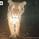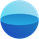1747 views
Get a clear picture of trend direction by visualizing RSI levels on your charts.
Green = buy zone, red = sell zone.

The RERSI was first developed by Giorgos Siligardos in the June 2003 issue of Stocks and Commodities Magazine. RERSI plots lines on the price chart that reflect levels of the RSI .

Modified from Original implementation by HPotterjaggedsoft
@jaggedsoft, been scrolling back and works awesome for some things on 15min too! My new favourite indicator. Would have saved me from many FOMO chasing trades and helped confirm entry points. Big question please sir is how to run/set alerts on this if at all possible? They don't seem to fire on TradingView. Many thanks for this work.Hi jaggedsoft : ) Great indicator! Thanks for sharing it with us.
If you have the time, would you be interested in converting this piece of code from your indicator to V4 for me?

reRSI(lenMA, Length) =>
ExpPer = 2 * lenMA - 1
K = 2 / (ExpPer + 1)
AUC = 0.0
AUC := iff(src > src, K * (src - src) + (1 - K) * nz(AUC, 1), (1-K) * nz(AUC, 1))
ADC := iff(src > src, (1-K) * nz(ADC, 1), K * (src - src) + (1 - K) * nz(ADC, 1))
nVal = (lenMA - 1) * (ADC * Length / (100 - Length) - AUC)
nRes = iff(nVal >= 0, src + nVal, src + nVal * (100 - Length) / Length)

nl1 = reRSI(lenMA, 50)

I'm working on a script that has a variety of different moving averages, and I'm trying to add the above piece of code to it, but I'm new to pinecoding, and I'm not having any luck getting it to work. I'm pretty sure it's not working because the script I'm trying to add it to is in V4 and the above code is in V3...On second thought, the trouble might be coming more from the format that the different scripts are in, rather than just the V3 vs V4 issue? Here's an example of how the various moving averages are listed in the code that I'm trying to add your piece of script to.

ma(type, src, len) =>
float result = 0
if type=="SMA" // Simple
result := sma(src, len)
if type=="EMA" // Exponential
result := ema(src, len)
if type=="DEMA" // Double Exponential
e = ema(src, len)
result := 2 * e - ema(e, len)
if type=="TEMA" // Triple Exponential
e = ema(src, len)
result := 3 * (e - ema(e, len)) + ema(ema(e, len), len)
if type=="JMA" // Jurik
phaseRatio = jurik_phase < -100 ? 0.5 : jurik_phase > 100 ? 2.5 : jurik_phase / 100 + 1.5
beta = 0.45 * (len - 1) / (0.45 * (len - 1) + 2)
alpha = pow(beta, jurik_power)
jma = 0.0
e0 = 0.0
e0 := (1 - alpha) * src + alpha * nz(e0)
e1 = 0.0
e1 := (src - e0) * (1 - beta) + beta * nz(e1)
e2 = 0.0
e2 := (e0 + phaseRatio * e1 - nz(jma)) * pow(1 - alpha, 2) + pow(alpha, 2) * nz(e2)
jma := e2 + nz(jma)
result := jma

Anyways, thanks again for all the awesome indicators you've generously shared with us. I'm sure I speak for a ton of people when I say it's It's very much appreciated!